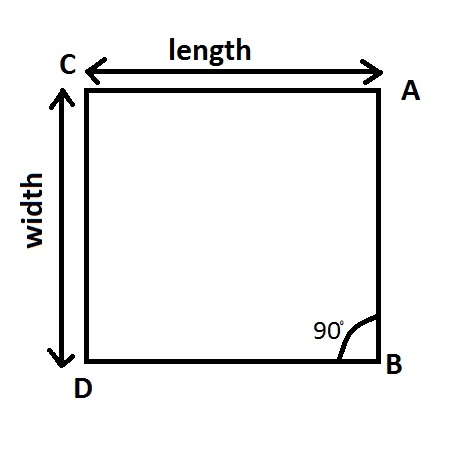# The length of a rectangular swimming pool is 2x-1 meters and the width is x+2 meters. (a) Write...

## Question:

The length of a rectangular swimming pool is {eq}2x-1 {/eq} meters and the width is {eq}x+2 {/eq} meters. (a) Write a polynomial {eq}A(x) {/eq} that represents the area. (b) Find {eq}A(5) {/eq}.

## Rectangle

A rectangle is a two dimensional figure with two pair of equal straight sides that are all at right angles, i,e. {eq}90^o. {/eq}

The image shown below is a figure of rectangle ABCDFollowing are the properties of a rectangle:

Area: length * width

Perimeter: 2* (length + width)

Number of Sides: 4

Number of edges: 4

Sum of angles : 360

The dimension of rectangular swimming pool is {eq}(2x-1) \times (x+2) {/eq}

Part a.) Area of the rectangular pool is:

$$Area =length \times width$$

$$A(x) =(2x-1)*(x+2)$$

$$A(x) =2x^2+4x- x-2$$

$$A(x) =2x^2+3x-2$$

Part b.) Area at {eq}x=5 {/eq}

$$A(x) =2x^2+3x-2$$

$$A(5) =2(5)^2+3*5-2$$

$$A(5) =2*25+15-2$$

$$A(5) =50+13$$

$$A(5) =63\ m^2$$

Area of the rectangular swimming pool at {eq}A(5) {/eq} is {eq}63\ m^2. {/eq}